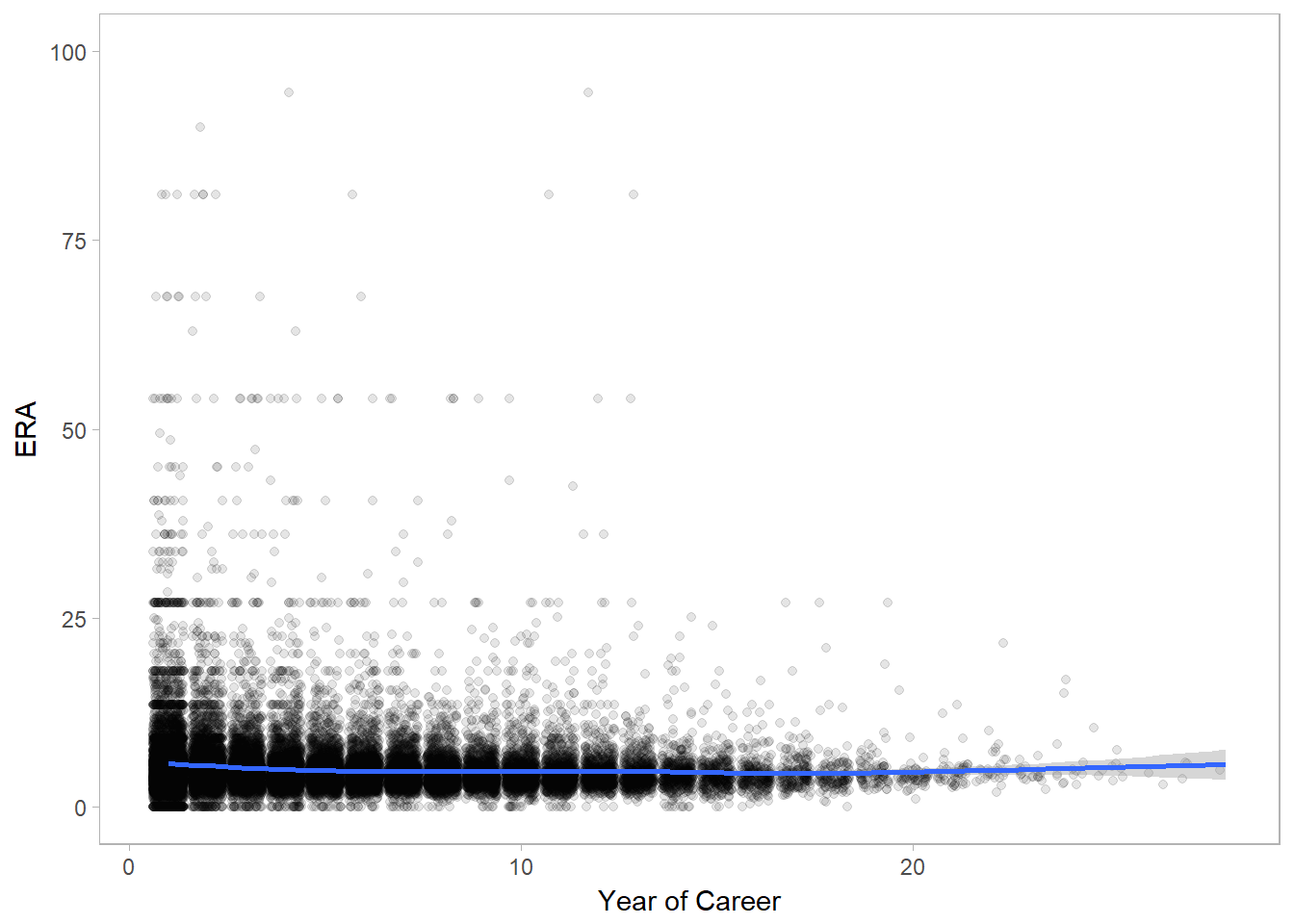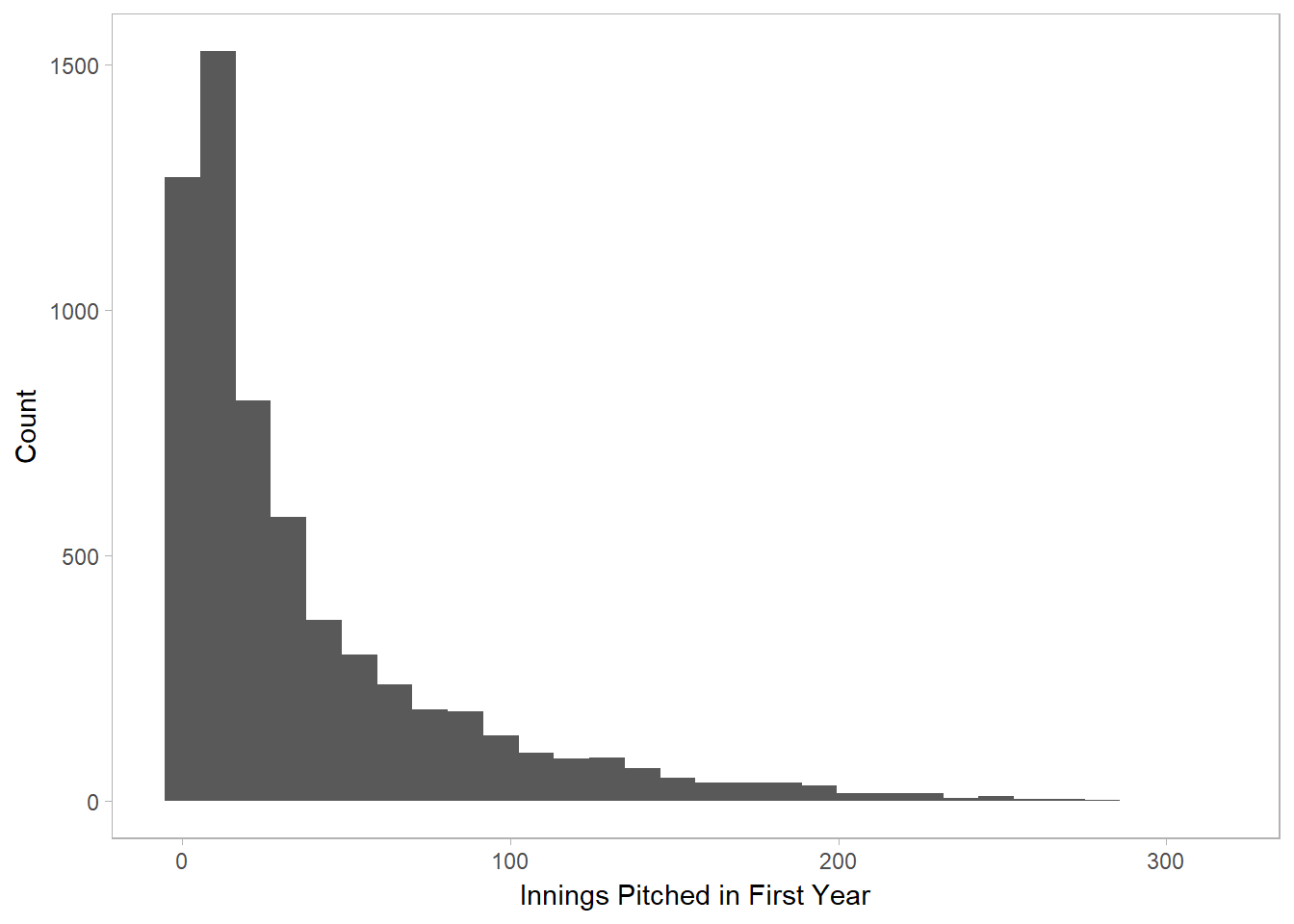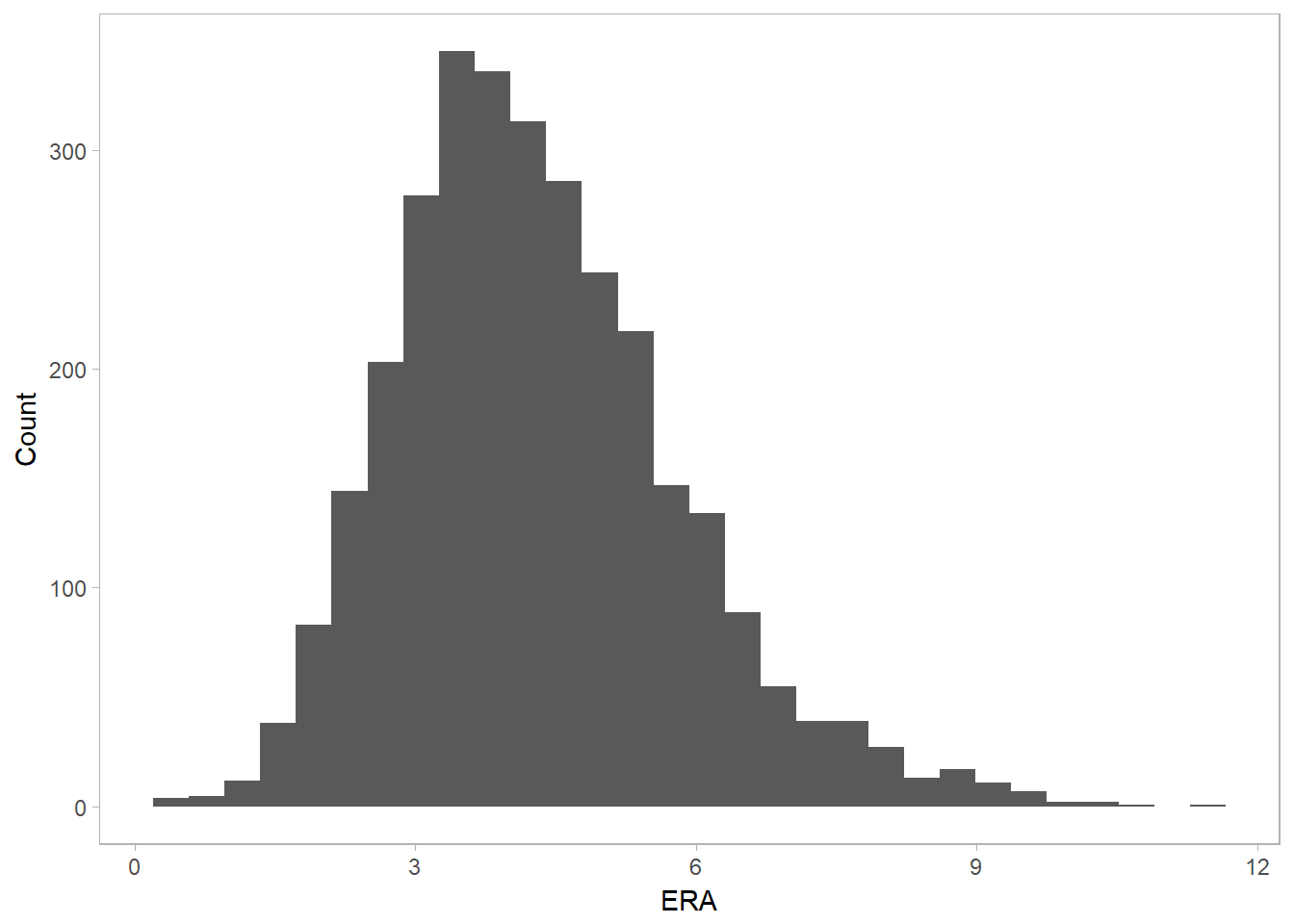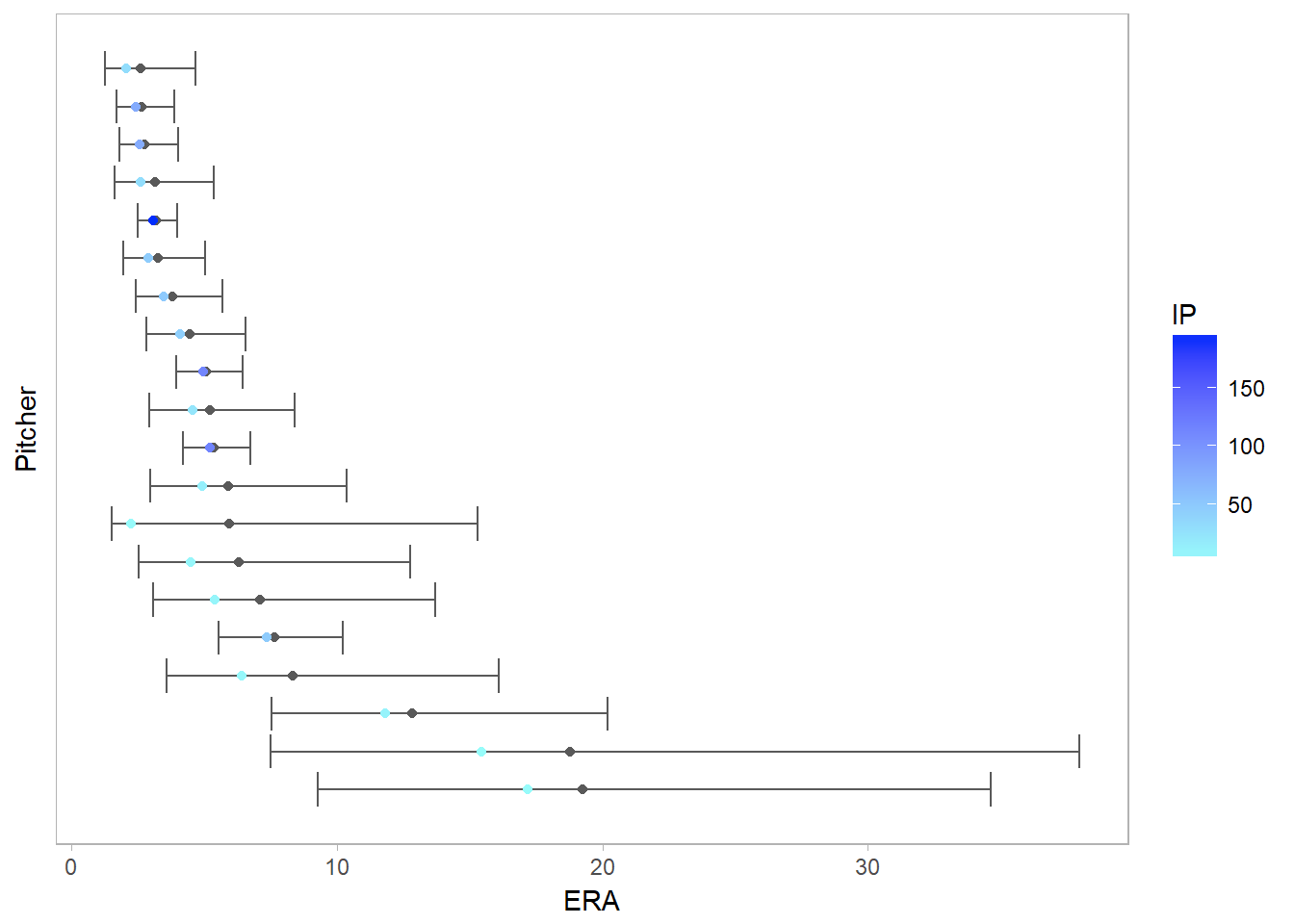#### By Jeff Hughes (July 22, 2017)

Predicting things with varying amounts of data is a common problem. If you are comparing two products, one of which has a 4.5 / 5 rating based on 3 reviews, and another with a 4.0 / 5 rating based on 1200 reviews, which is the better product? On the one hand, in an objective sense, 4.5 is higher than 4.0. On the other hand, one of those ratings is based on much less data. Maybe the three people who reviewed the first product all just happened to love it. Or maybe those ratings were given by friends of the seller!

In cases like these, it is important to weigh the data itself (i.e., the rating) by the amount or quality of the data you have. Implicit in this is that when we have little data, we should be more uncertain about the true value—and as such, we need to incorporate this uncertainty into our predictions. This is a situation where Bayesian models are an excellent choice.

So let’s talk about one of these scenarios in baseball. I am not a baseball fanatic. When I was young, I did enjoy keeping up with baseball. But to be honest, I think I got more enjoyment from updating the team statistics on my Excel spreadsheet than I did actually watching the games.1 But I haven’t really followed baseball in 15 years or more. That said, baseball is a game for nerds. There are lots of great statistics you can use in baseball—and people in the stands who actually sit there meticulously keeping track of them. The following post is inspired by a post on baseball batting averages by David Robinson on empirical Bayes, but applied to a different context.

## The Problem

Let’s say we want to evaluate major league pitchers. One key statistic used for pitchers is their ERA: Earned Run Average. This is calculated by taking the number of earned runs2 they allowed while pitching and dividing it by the number of innings pitched.3 This value is then multiplied by 9 to extend the average over 9 innings. So essentially, the lower a pitcher’s ERA, the better a pitcher they are—they allow the other team to get fewer runs.

Pitchers already vary in the number of innings they pitch. Like the example of product reviews, an ERA calculated over fewer innings is likely to be a more unstable estimate of a pitcher’s true ability. So this would be a great case for using a Bayesian approach. However, let’s make it a bit more challenging still. What I’d like to do is take a pitcher’s ERA over just their first year of pitching, and see how well we can predict what their overall ERA will be over the rest of their entire career.4 This is quite a challenge—how accurately can we predict a pitcher’s career based on the dozen or so innings they pitched as a rookie? Well, let’s try it and find out.

## Exploring the Data

I first load some packages and the data. You can see the code for this analysis by clicking on the buttons to the right, or go to the top of the page on the right and select “Show All Code”. The raw code is also available on Github. I’m using Sean Lahman’s baseball database, an excellent source of data with statistics all the way from 1871 to 2016. However, I’m going to select only data from 1945 onwards, as the game itself has evolved in ways that might introduce a lot more complexity. That still gives us plenty of data to work with.

library(knitr)
library(ggplot2)
library(dplyr)
theme_set(theme_light() + theme(panel.grid.major=element_blank(), panel.grid.minor=element_blank()))

pitching <- pitching %>%
filter(yearID >= 1945, IPouts > 0) %>%
group_by(playerID) %>%
mutate(year=yearID - first(yearID) + 1) %>%
ungroup()

Below you can see a plot of pitchers’ ERAs over each year of their career. You can see a couple things: First off, most pitchers have a career spanning less than 20 years. Second, while the average ERA stays relatively stable (as noted by the loess line in blue), there is much more variability in the early years. However, this may be due in part to calculating averages over fewer innings pitched. It’s easy to have an absurdly high (or low) ERA if you’ve only pitched a single inning!

ggplot(pitching, aes(x=year, y=ERA)) +
geom_jitter(alpha=.1) +
geom_smooth() +
ylim(0, 100) +
labs(x='Year of Career') +
theme(panel.grid.major=element_blank(),
panel.grid.minor=element_blank())Now I’ll pull out the first year of data for each pitcher, and calculate their ERA over that year. On average, pitchers pitched about 40 innings in their first year, plus or minus around 46. However, there’s some skew to the data, so the graph below might give you a better picture.

first_year <- pitching %>%
filter(year == 1)

first_year <- first_year %>%
group_by(playerID) %>%
summarize(ER=sum(ER), IP=(sum(IPouts) / 3)) %>%
mutate(ERA=9 * ER/IP)

first_year <- master %>%
select(playerID, nameFirst, nameLast) %>%
inner_join(first_year, by='playerID') %>%
arrange(desc(ERA))

cat('Mean:', mean(first_year$IP), '\nStandard Deviation:', sd(first_year$IP))
## Mean: 38.39035
## Standard Deviation: 46.57904
ggplot(first_year, aes(x=IP)) +
geom_histogram() +
labs(x='Innings Pitched in First Year', y='Count')So let’s make sure we actually have an issue here first. If we have an issue with minimal data for some players, we should see that the people with really high or really low ERAs tend to have little data (few IP). People with more data should tend to fall somewhere in the middle instead of at the extremes. Here are the 10 pitchers with the highest ERA:

first_year %>%
select(nameFirst, nameLast, ER, IP, ERA) %>%
kable(
row.names=FALSE,
col.names=c('First Name', 'Last Name', 'ER', 'IP', 'ERA'),
align='llccc',
digits=c(NA, NA, 0, 2, 1))
First Name Last Name ER IP ERA
Joe Cleary 7 0.33 189.0
Jeff Ridgway 7 0.33 189.0
Tom Qualters 6 0.33 162.0
Fritz Fisher 4 0.33 108.0
Gene Bearden 3 0.33 81.0
Ricky Pickett 6 0.67 81.0
Terry Wilshusen 3 0.33 81.0
Manny Alexander 5 0.67 67.5
Larry Harlow 5 0.67 67.5
Akeel Morris 5 0.67 67.5

And here are the pitchers with the lowest ERA:

first_year %>%
select(nameFirst, nameLast, ER, IP, ERA) %>%
tail(n=10) %>%
kable(
row.names=FALSE,
col.names=c('First Name', 'Last Name', 'ER', 'IP', 'ERA'),
align='llccc',
digits=c(NA, NA, 0, 2, 1))
First Name Last Name ER IP ERA
Fred Wenz 0 1.00 0
Ron Willis 0 3.00 0
Bill Wilson 0 1.00 0
Glenn Wilson 0 1.00 0
Josh Wilson 0 1.00 0
Dewayne Wise 0 1.67 0
Jason Wood 0 1.00 0
Ray Yochim 0 1.00 0
Todd Zeile 0 1.00 0

As you can see, they all have very little data. One pitcher, Jeff Zaske, surprisingly pitched 5 innings without an earned run, but the rest are around 1 inning or less. Obviously, this isn’t too helpful for figuring out who the best pitchers are.

## Constructing the Bayesian Model

To solve this problem, I am going to use empirical Bayes to estimate a “true 1st-year ERA” for pitchers. This is then applied to all pitchers in our data set to essentially “pull in” pitchers with little data toward the mean. The general idea is this: If we had absolutely no information about a pitcher, our best guess would be the mean. If we have just a little information about a pitcher, we can use that information, but we should still weight that along with the mean so that our predictions get pulled in closer to the mean. The more data we have, the more weight we give to that data and the less weight we give to the mean in our prediction.

ERA can be considered a rate variable, modelled by a Poisson process where earned runs occur at a certain rate per inning pitched. The prior probability for the rate can be modelled by a Gamma distribution, and because I’m using an empirical Bayes approach, I’m going to use the data to determine the hyperparameters of that Gamma distribution. I’m actually going to grab a subset of the data in order to provide a better estimate—I’ll restrict the data to those who have pitched more than 20 innings. This just ensures that we get rid of some of those extremes at either end. Here’s the distribution for those 20-inning-plus pitchers:

first_year_filtered <- first_year %>%
filter(IP > 20)
ggplot(first_year_filtered, aes(x=ERA)) +
geom_histogram() +
labs(y='Count')We can take the mean and variance of that distribution to determine the shape ($$\alpha_0$$) and rate ($$\beta_0$$) for our Gamma distribution. (If you’re not familiar with Bayesian approaches, that’s fine. The bottom line is that I’m using the data to figure out a general “average” ERA.)

cat('Mean:', mean(first_year_filtered$ERA), '\nVariance:', var(first_year_filtered$ERA))
## Mean: 4.314126
## Variance: 2.314021
# shape / rate = mean
# shape / rate^2 = variance
# therefore, mean*rate - variance*rate^2 = 0
# we then solve for the roots of this equation to get the rate
rate <- as.numeric(polyroot(c(-var(first_year_filtered$ERA), mean(first_year_filtered$ERA))))
shape <- mean(first_year_filtered$ERA) * rate If we assume the likelihood is generated from a Poisson distribution, then Gamma and Poisson are what are known as conjugate priors, which means we get another Gamma distribution out at the end. That just makes the math a little easier; if we wanted to model this differently, you could also use Markov Chain Monte Carlo (MCMC) methods to sample from the posterior distribution. I’ll return to that at the end when talking about some ways to extend this example. However, right now here’s what I’m doing: I just calculated a prior Gamma distribution above based on the data. Then, for each pitcher in the data set, I combine the prior distribution and their actual data to create a posterior Gamma distribution for each pitcher. The conjugate priors make this relationship nice and simple; the posterior shapes and rates for each pitcher ($$i$$) are calculated as follows: $$\alpha_i = \alpha_0 + ER_i$$ $$\beta_i = \beta_0 + IP_i$$ This means we get a full probability distribution for each player, giving us the likely values for each player’s true ERA. I could leave that there if I wanted to, but given that I want to use this to predict their future career ERA, it’s useful to also grab some “best estimate” for each player. Below I calculate the median value; however, you could just as easily calculate the mean, or some other estimate of your choosing. first_year$post_ERA_shape <- shape + first_year$ER first_year$post_ERA_rate <- rate + first_year$IP first_year$post_ERA_mdn <- qgamma(.5, shape=first_year$post_ERA_shape, rate=first_year$post_ERA_rate) * 9

first_year <- first_year %>%
arrange(desc(post_ERA_mdn))

After sorting by this median estimate, then, here are the estimates for the pitchers with the highest ERA:

first_year %>%
select(nameFirst, nameLast, ER, IP, ERA, post_ERA_mdn) %>%
kable(
row.names=FALSE,
col.names=c('First Name', 'Last Name', 'ER', 'IP', 'ERA', 'Median Est. ERA'),
align='llcccc',
digits=c(NA, NA, 0, 2, 1, 1))
First Name Last Name ER IP ERA Median Est. ERA
Joe Cleary 7 0.33 189.0 93.0
Jeff Ridgway 7 0.33 189.0 93.0
Tom Qualters 6 0.33 162.0 82.6
Fritz Fisher 4 0.33 108.0 61.9
Ricky Pickett 6 0.67 81.0 59.7
Manny Alexander 5 0.67 67.5 52.2
Larry Harlow 5 0.67 67.5 52.2
Akeel Morris 5 0.67 67.5 52.2
Jay Sborz 5 0.67 67.5 52.2
Gene Bearden 3 0.33 81.0 51.6

And here are the pitchers with the lowest estimates:

first_year %>%
select(nameFirst, nameLast, ER, IP, ERA, post_ERA_mdn) %>%
tail(n=10) %>%
kable(
row.names=FALSE,
col.names=c('First Name', 'Last Name', 'ER', 'IP', 'ERA', 'Median Est. ERA'),
align='llcccc',
digits=c(NA, NA, 0, 2, 1, 1))
First Name Last Name ER IP ERA Median Est. ERA
Mike Hinckley 0 13.67 0.0 1.3
Jeremy Hernandez 0 14.33 0.0 1.2
Joel Johnston 1 22.33 0.4 1.2
Dave Ford 0 15.00 0.0 1.2
Bob McClure 0 15.33 0.0 1.1
Joba Chamberlain 1 24.00 0.4 1.1
Mike Norris 0 16.67 0.0 1.0
Fernando Valenzuela 0 17.67 0.0 1.0
Karl Spooner 0 18.00 0.0 1.0
Kevin Siegrist 2 39.67 0.5 0.9

Notice that for the highest ERAs, the estimates have been brought down, while for the lowest ERAs, the estimates have been pushed up. They’ve all been “regressed toward the mean” at least in part. But also notice that the predictions for the best pitchers (low ERA) are no longer based on people with practically no data. We’ve ended up with better estimates, because those pitchers who had 0 ERA with practically no data have been pushed up toward the mean. (On the high end, this doesn’t work so well, because ERA has a lower limit of 0, but no upper limit. But we’ve still brought in those high estimates quite a bit.)

Below I’ve taken a random sample of 20 pitchers in the data, and graphed their actual first-year ERA with blue dots. The intensity of the blue indicates how much data we had (i.e., how many innings they pitched). Alongside that, I’ve also graphed our Bayesian estimates for each pitcher, with a gray dot at the median, and error bars indicating the 95% credible interval (the middle 95% of the probability distribution). Notice that the pitchers for which we had little data (light blue) have had their estimates pulled toward the mean to a greater extent, and the probability distributions are very wide; for pitchers for which we had more data (dark blue), the estimate does not change as much, and the error bars are much smaller. In other words, we can be more confident about their true ERA.

set.seed(123)
sample_ERA <- first_year[sample(1:nrow(first_year), 20), ]
sample_ests <- data.frame(
ERA=sample_ERA$ERA, IP=sample_ERA$IP,
lower=qgamma(.025, shape=sample_ERA$post_ERA_shape, rate=sample_ERA$post_ERA_rate)*9,
median=qgamma(.5, shape=sample_ERA$post_ERA_shape, rate=sample_ERA$post_ERA_rate)*9,
upper=qgamma(.975, shape=sample_ERA$post_ERA_shape, rate=sample_ERA$post_ERA_rate)*9
)
sample_ests <- sample_ests %>%
arrange(desc(median)) %>%
mutate(k=1:20)

ggplot(sample_ests, aes(y=k)) +
geom_point(aes(x=median), color='#595959') +
geom_errorbarh(aes(x=median, xmin=lower, xmax=upper), color='#595959') +
geom_point(aes(x=ERA, color=IP)) +
labs(x='ERA', y='Pitcher') +
theme(axis.text.y=element_blank(), axis.ticks.y=element_blank(), panel.grid.major.y=element_blank(),
panel.grid.minor.y=element_blank())## Predicting Career ERA

Okay, the real test of this model is to use it to predict pitchers’ ERA over the rest of their career. As I said earlier, this is a challenging task, given that I am trying to use people’s performance in their rookie year to predict their performance up to 20 years later! This is also a relatively simplistic model: I’m implicitly assuming that pitchers’ performance does not change over their career; that there is no difference between pitchers across the decades, across teams, etc. The only bits of information I’ve used is the ER in their first year, and the number of innings they pitched.

Below I’ve calculated the career ERA for all pitchers in the data set, then provided two estimates. Our baseline is the “naive estimate”, which simply uses their actual ERA from their first year as the prediction. The comparison is the “Bayesian estimate”, which is the median value we estimated for each pitcher. In the table below, I have calculated the squared error between the actual career ERA and these two estimates, and then sorted it based on the difference in the squared error.

Note that one complication here is that in some cases, the career ERA score is not based on much more data than the first-year ERA score was! Because there is such variability in the length of career, some pitchers’ career ERAs might not be much of a “true score” and might be very noisy estimates. I do try to restrict this a little by restricting to those who had pitched at least five innings after their first year, but in some cases the values to predict have almost as much noise as the predictions. However, this is more of a proof of concept (I don’t recommend using this simplistic a model for Sabermetrics!), so I’d rather err on the side of including more data in the career scores.

First, here are the top 20 cases, for which the Bayesian estimate did very well compared to baseline:

career <- pitching %>%
filter(year != 1)

career <- career %>%
group_by(playerID) %>%
summarize(ER_crr=sum(ER), IP_crr=(sum(IPouts) / 3)) %>%
filter(IP_crr > 5) %>%
mutate(ERA_crr=9 * ER_crr/IP_crr)

career <- career %>%
inner_join(first_year, by='playerID') %>%
arrange(desc(ERA_crr))

career$naive_se <- (career$ERA_crr - career$ERA)^2 career$bayes_se <- (career$ERA_crr - career$post_ERA_mdn)^2
career$diff <- career$naive_se - career\$bayes_se

career %>%
arrange(desc(diff)) %>%
select(nameFirst, nameLast, ERA_crr, IP_crr, ERA, IP, post_ERA_mdn, naive_se, bayes_se, diff) %>%
digits=c(NA, NA, 1, 2, 1, 2, 1, 2, 2, 2))